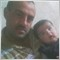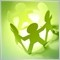665

how they calculate the floating spread each tick ? for each broker is there any math equations to achieve that ? if you test too many robots on strategy tester absolutely you notice the big difference between real market environments and your tester's !!! the main difference is due to floating spread but if we know the equation then we could get the real Ask price then the real markets environments could be applied am i wrong   ???36

Prices are generated by the exchanging operations in the market. The price you see on the chart is the only real exchange price which is the Bid. Borkers artificially creates the Ask in order to gain their part, but when the market moves very fast, the Bid run away faster than every attempt to recalculate the Ask, so you can find the Ask calculated for a Bid price of some milliseconds before and the spread could be larger (or sometimes smaller) than the average one decided by the broker. That's not an equation, it's an unpredictable behavior of the market and must be considered as a random variable. Your system needs to be loose enough in order to make it negligible.19654

In addition, brokers artificially increase the spread at end of day (5pm ET) and before news events. On my broker, GBPJPY averages 2.6 but averages 12.2 at EOD.4106

floating spread for eurusd: